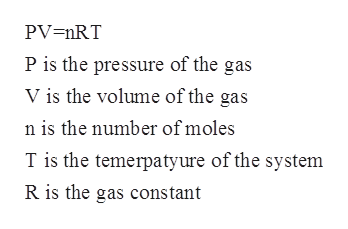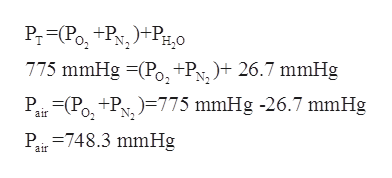# A 355 cm^3 sample of air saturated with water vapor at 27 degrees celsius generates a total pressure of 775 mmHg. How many grams of O2 are present in the sample, given that water has a vapor pressure of 26.7 mmHg and air can be assumed to be 20% O2 and 80% N2?

Question
3 views

A 355 cm^3 sample of air saturated with water vapor at 27 degrees celsius generates a total pressure of 775 mmHg. How many grams of O2 are present in the sample, given that water has a vapor pressure of 26.7 mmHg and air can be assumed to be 20% O2 and 80% N2?

check_circle

Step 1

The number of moles for a given chemical species refers to the ratio of mass to the molar mass. The mathematical formula which is used to calculate the number of moles is as follows:

Step 2

The ideal gas law which is also known as the equation of state is an equation of state of a hypothetical ideal gas. The equation can be written as follows:help_outlineImage TranscriptionclosePV=nRT P is the pressure of the gas V is the volume of the gas n is the number of moles T is the temerpatyure of the system R is the gas constant fullscreen
Step 3

The volume of the sample of the air is given to be 355 cm3=0.355 L.

T=27oC=300.15 K

Ptotal=775 mmHg

Pwater= 26.7 mmHg

...help_outlineImage TranscriptioncloseР. -Ро, "Ру, -Рно 775 mmHg Po,+P,)+ 26.7 mmHg P -(Ро, +Ру, )-775 mmHg -26.7 mmHg Р. -748.3 mmHg air air fullscreen

### Want to see the full answer?

See Solution

#### Want to see this answer and more?

Solutions are written by subject experts who are available 24/7. Questions are typically answered within 1 hour.*

See Solution
*Response times may vary by subject and question.
Tagged in

### Physical Chemistry